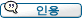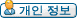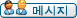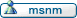글쓴이 메시지
박창범

가입: 2007년 3월 21일
올린 글: 4올려짐: 2007년4월11일 12:42    주제: 식을 평가하면 이렇게 되는건가요?아래 처럼 정의가 되었다고 하면 (define nil ()) (define (link x lst) (lambda (f) (f x lst))) (define (first lst) (lst (lambda (x y) x))) (define (rest lst) (lst (lambda (x y) y))) (define empty? null?) 이를 실행시에 (first (link 1 nil)) => (first (lambda (f) (f 1 nil))) => ((lambda (f) (f 1 nil)) (lambda (x y) x)) => ((lambda (x y) x) 1 nil) => 1 순서로 평가가 되는것인지요? 아니면 => ((link 1 nil) (lambda (x y) x)) => ((lambda (f) (f 1 nil)) (lambda (x y) x)) => ((lambda (x y) x) 1 nil) => 1 이렇게 평가가 되는건지요? 복잡하네요.공순호

가입: 2005년 9월 29일
올린 글: 363
위치: 302동 312-2호올려짐: 2007년4월12일 6:54    주제:좋은 질문이네요.인용: SICP, 1.1.3 Evaluating Combinations One of our goals in this chapter is to isolate issues about thinking procedurally. As a case in point, let us consider that, in evaluating combinations, the interpreter is itself following a procedure. * To evaluate a combination, do the following: 1. Evaluate the subexpressions of the combination. 2. Apply the procedure that is the value of the leftmost subexpression (the operator) to the arguments that are the values of the other subexpressions (the operands).

 인용: SICP, 1.1.5 The Substitution Model for Procedure Application Applicative order versus normal order According to the description of evaluation given in section 1.1.3, the interpreter first evaluates the operator and operands and then applies the resulting procedure to the resulting arguments. This is not the only way to perform evaluation. An alternative evaluation model would not evaluate the operands until their values were needed. Instead it would first substitute operand expressions for parameters until it obtained an expression involving only primitive operators, and would then perform the evaluation. . . . This alternative ``fully expand and then reduce'' evaluation method is known as normal-order evaluation, in contrast to the ``evaluate the arguments and then apply'' method that the interpreter actually uses, which is called applicative-order evaluation. It can be shown that, for procedure applications that can be modeled using substitution (including all the procedures in the first two chapters of this book) and that yield legitimate values, normal-order and applicative-order evaluation produce the same value. (See exercise 1.5 for an instance of an ``illegitimate'' value where normal-order and applicative-order evaluation do not give the same result.) Lisp uses applicative-order evaluation, partly because of the additional efficiency obtained from avoiding multiple evaluations of expressions such as those illustrated with (+ 5 1) and (* 5 2) above and, more significantly, because normal-order evaluation becomes much more complicated to deal with when we leave the realm of procedures that can be modeled by substitution. On the other hand, normal-order evaluation can be an extremely valuable tool, and we will investigate some of its implications in chapters 3 and 4.16

읽어보시면 답을 아실 수 있을겁니다.
_________________
- soon@ropas이전 글 표시: 모든 글1일7일2주1달3달6달1년 오래된 글 먼저새로운 글 먼저
 시간대: GMT + 9 시간(한국) 페이지 1 중 1

 건너뛰기: 게시판 선택 010.142 Basics in Computing----------------010.142 Basics in Computing (Spring 2006) 400.002 Engineering Mathematics II----------------400.002 Engineering Mathematics II (Fall 2005) 4190.210 Principles of Programming----------------4190.210 Principles of Programming (Fall 2014)4190.210 Principles of Programming (Fall 2013)4190.210 Principles of Programming (Fall 2012)4190.210 Principles of Programming (Fall 2011)4190.210 Principles of Programming (Fall 2010)4190.210 Principles of Programming (Fall 2009)4190.210 Principles of Programming (Fall 2008)4190.210 Principles of Programming (Spring 2007)4190.210 Principles of Programming (Spring 2006) 4190.310 Programming Languages----------------4190.310 Programming Languages (Spring 2022)4190.310 Programming Languages (Spring 2021)4190.310 Programming Languages (Fall 2019)4190.310 Programming Languages (Fall 2018)4190.310 Programming Languages (Fall 2017)4190.310 Programming Languages (Fall 2015)4190.310 Programming Languages (Fall 2014)4190.310 Programming Languages (Fall 2013)4190.310 Programming Languages (Fall 2012)4190.310 Programming Languages (Fall 2011)4190.310 Programming Languages (Spring 2011)4190.310 Programming Languages (Fall 2010)4190.310 Programming Languages (Fall 2009)4190.310 Programming Languages (Fall 2008)4190.310 Programming Languages (Fall 2007)4190.310 Programming Languages (Fall 2006)4190.310 Programming Languages (Fall 2005) 4541.664A Program Analysis----------------4541.664A Program Analysis (Spring 2017)4541.664A Program Analysis (Spring 2016)4541.664A Program Analysis (Spring 2015)4541.664A Program Analysis (Spring 2014)4541.664A Program Analysis (Spring 2013)4541.664A Program Analysis (Spring 2011)4541.664A Program Analysis (Spring 2010)4541.664A Program Analysis (Spring 2009)4541.664A Program Analysis (Fall 2008)4541.664A Program Analysis (Spring 2007)4541.664A Program Analysis (Spring 2006) 027.013 Computational Civilization----------------046.016 Computational Civilization (Spring 2018)046.016 Computational Civilization (Spring 2017)046.016 Computational Civilization (Spring 2016)046.016 Computational Civilization (Fall 2015)046.016 Computational Civilization (Spring 2015)046.016 Computational Civilization (Spring 2014)027.013 Computational Civilization (Spring 2013)027.013 Computational Civilization (Fall 2012) L444.200 Computational Thinking and Practice----------------L444.200 Computational Thinking and Practice (Fall 2017)
새로운 주제를 올릴 수 없습니다
답글을 올릴 수 없습니다
주제를 수정할 수 없습니다
올린 글을 삭제할 수 없습니다
투표를 할 수 없습니다

Powered by phpBB 2.0.21-7 (Debian) © 2001, 2005 phpBB Group
Translated by kss & drssay